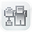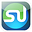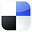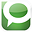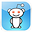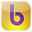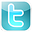4-Part Training on Two of
Excel's Most Versatile and
Complicated Statistical
Functions:

VLOOKUP & Pivot Tables

First Name

Email

 What  Customers Are Saying  About  the Excel Statistical Master "We just started building statistical excel spreadsheets for our direct mail and online marketing campaigns, I purchased Excel Statistical Master to help fill in some of the blanks. Little did I know, this book has everything I could ever want to know about business statistics. Easy to follow and written so even a child could understand some of the most complex statistical theories. Thanks Mark!" Brandon Congleton Marketing Director www.worldprinting.com "After years of searching for a simplified statistics book, I found the Excel Statistical Master. Unlike the indecipherable jargon in the countless books I have wasted money on, the language in this book is plain and easy to understand. This is the best \$40 I have ever spent. " Mahdi Raghfar "I really like the Excel Statistical Master. It is incredibly useful. The explanations and videos in the manual are excellent. It has really made my work with statistics a LOT easier. I'm really glad that I came across the manual. If you're a student of business statistics, this e-manual is worth WAY more it's priced. I will use your manual as a reference for my MBA course this summer." Dr. Yan Qin Co-Director Nankai-Grossman Center for Health Economics and Medical Insurance
Excel
STATISTICAL
Master

The Binomial Distribution

Clear and Complete - WITH LOTS OF SOLVED PROBLEMS

# Binomial Distribution

Lots of Worked-Out, Easy-To-Understand, Graduate-Level Problems  --->
( Scroll Down and Take a Look ! )

Introduction

The Binomial Distribution is one of the most valuable and commonly used statistical tools. The Binomial Distribution is used whenever a process has only two possible outcomes. For example, the Binomial Distribution would be used to determine the probability of getting 4 heads on six flips of a coin. It could be used to determine the probability of 6 out of 10 people sampled voting Republican or the probability of 3 out of 20 people sampled having green eyes if the percentage of the overall population having green eyes or voting Republican is known.

## Basic Explanation of Binomial Distribution

The Binomial Distribution is applied to one sample of n trials taken from a much larger population. The Binomial Distribution will calculate the probability of the given number of successful outcomes in a given number number of trials if the proportion of the overall population having that outcome is known. The Binomial Distribution shows the distribution of the number of successes for a given number of trials if the following are true:

• Each trial has only two possible outcomes: "success" or "failure."

• The probability of a success, p,  of each trial is known and doesn't vary.

• The number of trials, n, is fixed

• Each trial is independent.

The Binomial Distribution can be completely described by 2 parameters. These are p (the probability of success in each trial) and n (the number of trials). If the above 4 conditions are met and also describe the behavior of count variable X, then X has a binomial distribution that can be abbreviated as:

B(n,p)

For example, the binomial distribution describing the outcome of 70 flips of a fair coin (50 / 50 chance of heads or tails on each flip) could be abbreviated as follows:

n = 70

p = 0.5

B(n,p) = B(70, 0.5)

As a general rule, the Binomial Distribution should only be applied to a sample if the population size is at least 10 times larger than the sample size ( n number of trials in the sample).

If samples are taken from a finite population, the Binomial Distribution can only be applied if the samples are being replaced. If the samples are not being replaced, the HyperGeometric Distribution can be applied.

If samples are taken from an infinite population, the Binomial Distribution can be applied whether or sampling is done with replacement..

## Bernoulli Trial

A Bernoulli Trial is a single random experiment whose outcome can have only one of two possibilities: "success" or "failure." An example of this would be one flip of a coin. This is the same as one trial in a sample of n random trials whose outcomes are independent and have only two possibilities.

## Bernoulli Process

A Bernoulli Process is a sequence of Bernoulli Trials. An example of a Bernoulli Process would be 5 flips of a coin. A "Bernoulli Process" is just another name for a single sample of n random trials whose outcomes are independent and have only two possibilities. These are exactly the samples that the Binomial Distribution is applied to.

## Bernoulli Distribution

A Bernoulli Distribution is just another name for the Binomial Distribution where the number of trials, n, equals 1. The term "Bernoulli Distribution" is not really an essential concept. The term is mentioned often in statistics books so it is presented here just to provide familiarity with it.

*******************************************************************

If you are trying to estimate a population proportion from the results of a sample proportion, see the module of this course entitled Confidence Intervals. Estimating a population proportion from a sample proportion is explained in the second half of that course module. In this module, we are doing almost the opposite of that. Here we are describing how to calculate the probability of a sample proportion if we know the population proportion.

*******************************************************************

## Binomial Distribution Parameters

Random Variable = X

This is the variable that will have 1 of 2 possible outcomes.

Count of Successes per n Trials = x

Population Proportion = p = proportion of a population having a certain outcome or characteristic. p also equals the probability of a certain outcome on 1 trial.

q = p - 1 = proportion of a population not having that outcome or characteristic. q also equals the probability of not getting a certain outcome on 1 trial.

If p = the probability of success,

then q = 1 - p = the probability of failure.

Sample Proportion = pavg = proportion of a sample having a certain outcome or characteristic

qavg = pavg - 1 = proportion of a sample not having that outcome or characteristic

Sample Size = n (This is the number of trials)

*******************************************************************

### Expected Sample Occurrence Parameters

Expected Sample Occurrence Mean = np
This is E(X) = the expected value of X (the count of successes in n trials)

Expected Sample Occurrence Variance = npq

Expected Sample Occurrence Standard Deviation = SQRT (npq)

*******************************************************************

### Expected Sample Proportion Parameters

Expected Sample Proportion = p

Expected Sample Proportion Variance = pq

Expected Sample Proportion Standard Deviation = SQRT (pq/n)

*******************************************************************

## Binomial Distribution Probability Density Function vs. Cumulative Distribution Function

Probability Density Function
= Pr (X = k)

The Probability Density Function (sometimes called the Probability Mass Function) calculates the probability of exactly k successes occurring in n trials. This is the probability that the count of successful outcomes, X, equals k  for n trials. For example, the Probability Density Function would be used to calculate the probability of exactly 4  heads in 6 flips of a coin.

Pr (X = k) = f(k; n, p) = n! / [ k! * ( n - k )! ] * pk * q(n-k)

 ( n ) =   nPk / k!  = n! / [ k! * ( n - k )! ] k

The above expression is known as the Binomial Coefficient and often referred to as "n chose k."  This is equal to the number of possible ways to choose k successes from n trials. This is equivalent to the total number of possible combinations of n objects taken k at a time simultaneously.

Example of Using the  Binomial Probability Density Function
to Solve a Problem

Problem: Calculate the probability of exactly 4 heads in 6 flips of a fair coin (50% of either heads or tails).

Probability of success in each trial = p = 0.5  ---> q = 1- p = 1 - 0.5  = 0.5

Number of trials = n = 6

Exact number of successes = k = 4

Probability of Success = p = 0.5

Pr (X = k) = f(k; n, p) = f(4; 6, 0.5)

= n! / [ k! * ( n - k )! ] * pk * q(n-k)

= 6! / [ 4! * ( 6 - 4 )! ] * (0.5)4 * (0.5)(6-4)

= 0.234

Pr (X = k) = 23.4%

The probability of exactly 4 heads in 6 flips of a fair coin is 23.4%.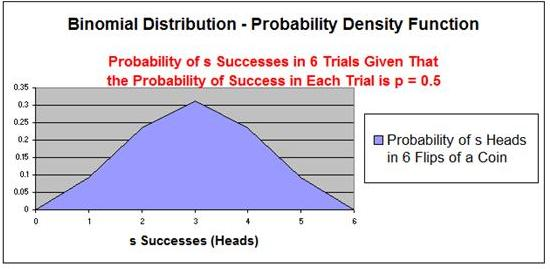## Binomial Distribution Cumulative Distribution Function

Cumulative Distribution Function = Pr (X ≤ k)

The probability of exactly k successes in n trials. This is the probability that the count of successful outcomes, X, equals any number between k and 0 for n trials. This equals the sum of the probabilities that X equals each number from 0 to k for n trials.

Example of Using the  Binomial Cumulative Distribution Function
to Solve a Problem

For example, the Cumulative Distribution Function would be used to calculate the probability of up to 4 heads in 6 flips of a coin.

Pr (X ≤ k) = Sum { Pr ( X = i ) } as i goes from 0 to k

Pr (X ≤ k) = Sum { n! / [ k! * ( n - k )! ] * pk * q(n-k) } as i goes from 0 to k

For example, Pr (X ≤ 3) = Pr (X=0) + Pr (X=1) + Pr (X=2) + Pr (X=3) + Pr(X=4)

Pr (X ≤ 4) = 0.016 + 0.094 + 0.234 + 0.312 + 0.234 = 0.890 = 89%

The probability of up to 4 heads in 6 flips of a fair coin is 89.0%.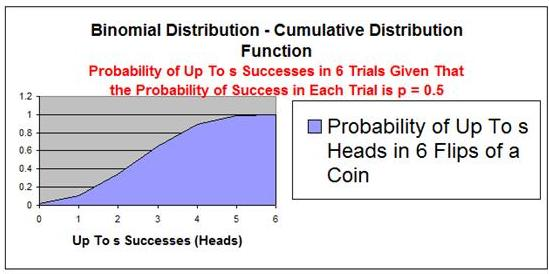## Using the Binomial Distribution to Estimate the Normal and Poisson Distributions

The Normal Distribution can be estimated using the Binomial Distribution if n is large and p and q are not too close to 0. If variable X is Binomially distributed and has parameters n (number of trials) and p (probability of success on each trial), for large values of n the distribution of X is approximately Normal. The mean and variance of the approximately Normal distribution of X are np and np(1-p). These are the same as the mean and variance of Binomial distribution that has parameters n and p.

The Normal approximation does not work well for small values of n. The Normal approximation should only be used if np>10 and np(1-p)>10.

For example, if n = 1000 and p = 0.4, then np = 400 and np(1-p) = 240

The Normal and Binomial Probability Density Functions should be very close for various values of the count variable X. This is demonstrated in the table below:

 Normal (X, µ, σ2) = n p np np(1-p) X Binomial (X, n, p) Normal (X, np, np(1-p) ) 1000 0.4 400 240 385 0.0162 0.0161 400 0.0257 0.0258 415 0.0160 0.0161

The Poisson Distribution can be estimated using the Binomial Distribution if n is large and p is small (less than 0.10). This approximation works well if n100 and np<10. The approximation is valid because the Binomial Distribution converges to the Poisson Distribution and n (the number of trials) approaches infinity and np remains fixed.

For example, if n = 100 and p = 0.03, then np = 3. If n ≥ 100  and  np < 10, then

the Poisson and Binomial Probability Density Functions should be very close for various values of the count variable X. This is demonstrated in the table below:

 Poisson (X, λ) = n p np X Binomial (X, n, p) Poisson (X, np) 100 0.03 3 1 0.0162 0.0161 2 0.0257 0.0258 3 0.0160 0.0161

The Binomial Distribution is a specific case of the more  general Multinomial Distribution

Problem 1

Problem 1: Probability of Getting a Certain Range of Successes for a Binomial Variable Trials

Problem: What is the probability of getting between 3 and 5 heads on 10 flips of a fair coin ( p = 0.50) ?

Probability of Success in Each Trial = p = 0.50

Number of Trials = n = 10

Maximum Number of Successes = k = 5

Minimum Number of Successes = k = 3

Probability of getting between 3 and 5 heads = Pr(X=3) + Pr(X =4) + Pr(X =5)

PR (X = 3) = f(k; n, p) = n! / [ k! * ( n - k )! ] * pk * q(n-k)

PR (X = 3) = f(3; 10, 0.50) = 100! / [ 3! * ( 10 - 3 )! ] * (0.50)3 * (0.50)(10-3)

PR (X = 3) = 0.1172 = 11.72%

PR (X = 4) = f(k; n, p) = n! / [ k! * ( n - k )! ] * pk * q(n-k)

PR (X = 4) = f(4; 10, 0.50) = 100! / [ 4! * ( 10 - 4 )! ] * (0.50)4 * (0.50)(10-4)

PR (X = 4) = 0.2050 = 20.50%

PR (X = 5) = f(k; n, p) = n! / [ k! * ( n - k )! ] * pk * q(n-k)

PR (X = 5) = f(5; 10, 0.50) = 100! / [ 5! * ( 10 - 5 )! ] * (0.50)5 * (0.50)(10-5)

PR (X = 5) = 0.2461 = 24.61%

Probability of getting between 3 and 5 heads = Pr(X=3) + Pr(X =4) + Pr(X =5)

Probability of getting between 3 and 5 heads = 11.72% + 20.50% + 24.61%

Probability of getting between 3 and 5 heads = 56.83%

There is a 56.83% probability of getting between 3 and 5 heads on 10 flips of a fair coin.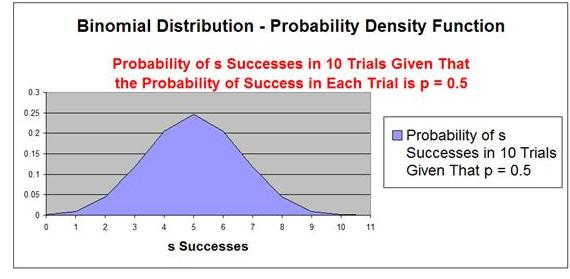This same problem is solved in the Excel Statistical Master with only 1 Excel formula. That's it. The Excel Statistical Master teaches you everything in step-by-step frameworks. You'll never have to memorize any complicated statisical theory.

Problem 2

Problem 2: Probability of Getting a Certain Range of Successes for a Binomial Variable Trials

Problem: What is the probability of that between 2 and 4 products out of 100 require service if 2% of all products require service?

Probability of Success in Each Trial = p = 0.02

Number of Trials = n = 100

Maximum Number of Successes = k = 4

Minimum Number of Successes = k = 2

Pr (2 ≤ X ≤ 4 ) = Pr ( X ≥ 2 ) AND Pr ( X ≤ 4 )

= Pr ( X ≤ 4 ) AND Pr ( X ≥ 2 )

= PR (X ≤ 4) - Pr ( X ≤ 2)

PR (X ≤ 4) = PR (X = 4) + PR (X = 3) + PR (X = 2) + PR (X = 1) + PR (X = 0)

PR (X ≤2)  = PR (X = 2) + PR (X = 1) + PR (X = 0)

PR (X ≤ 4)  - PR (X ≤2) = PR (X = 4) + PR (X = 3)

PR (X = 4) = f(k; n, p) = n! / [ k! * ( n - k )! ] * pk * q(n-k)

PR (X = 4) = f(4; 100, 0.02) = 100! / [ 4! * ( 100 - 4 )! ] * (0.02)4 * (0.98)(100-4)

PR (X = 4) = 0.0902 = 9.02%

PR (X = 3) = f(k; n, p) = n! / [ k! * ( n - k )! ] * pk * q(n-k)

PR (X = 3) = f(3; 100, 0.02) = 100! / [ 3! * ( 100 - 3 )! ] * (0.02)3 * (0.98)(100-3)

PR (X = 3) = 0.1823 = 18.23%

PR (X ≤ 4)  - PR (X ≤2) = PR (X = 4) + PR (X = 3)

PR (X ≤ 4)  - PR (X ≤2) = 9.02% + 18.23%
= 27.25%

There is a 27.25% probability that between 2 and 4 products out of 100 will need service if the probability of a product needing service is 2%.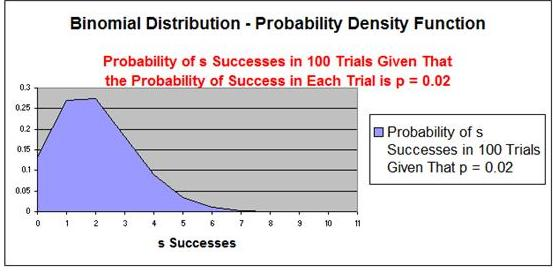This same problem is solved in the Excel Statistical Master with only 1 Excel formula. The Excel Statistical Master is the fastest way for you to climb the business statistics learning curve.

Problem 3

Problem 3: Finding Mean, Variance, and Standard Deviation Given p (Probability of Success) and n Number of Binomial Trial Occurrences.

Problem: A gambling machine makes a payout every 15 plays on average. The machine is played 40 times per hour on average. What is the mean number of payouts per hour and the variance and standard deviation in the number of hourly payouts? Assume that the occurrences of payout are binomially distributed

.

Probability of Success (Payout) in Each Trial = p = 1/15 = .06667

Probability of No Success (No Payout) in Each Trial = q = 1 - p = .93333

Number of Trials = n = 40

This problem uses Binomial formulas (Sample Occurrence Formulas) because what is being measured is the mean number of occurrences from a large number of individual samples that each have only two possible outcomes.

### Expected Sample Occurrence Parameters

Expected Sample Occurrence Mean = np = 40 * 0.06667 = 2.6667
This is E(X) = the expected value of X (the count of successes in n trials)

Expected Sample Occurrence Variance = npq = 2.6667 * 0.93333
= 2.4889

Expected Sample Occurrence Standard Deviation = SQRT (npq)
= SQRT(2.4889)
= 1.5776

The expected number (Mean) of payouts every hour is 2.6667 with a Variance of 2.4889 and a Standard Deviation of 1.5776.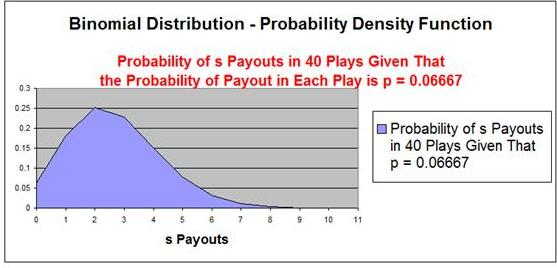Problem 4

Problem 4: Finding the Mean, Variance, and Standard Deviation of a Sampled Proportion (p) Given 1 Large Sample Containing n Subjects.

Problem: In a large sales force, the ratio of salespeople having college degrees to those not having degrees is 5 to 2. Determine the mean, variance, and standard deviation of the sampling distribution of the proportion of salespeople not having degrees in a random sample of 30 salespeople.

.

The problem uses proportion formulas because what is being measured is a proportion of a single large sample.

Proportion of salespeople not having degrees = p = 2/7 = 0.2857
Proportion of salespeople having degrees - q = 1 - p = 5/7 = 0.7143
Size of Sample = n = 30

### Expected Sample Proportion Parameters

Expected Sample Proportion = p = 0.2857

Expected Sample Proportion Variance = pq = (0.2857) * (0.7143)
= 0.2041

Expected Sample Proportion Standard Deviation = SQRT (pq/n)
=SQRT(0.2041 / 30)
= 0.0825

The expected Sample Proportion (Mean) of the proportion of degreed salespeople 0.2857 (28.57%) with a Variance of 0.2041 (20.41%) and a Standard Deviation of 0.0825 (8.25%).

Problem 5

Problem 5: Finding the probability of a certain number of sales for a specific number of customers given that probability of each customer purchasing, p (Probability of a sale), and n (Number of customers) is known.

Problem: A store has been tracking how many of their walk-in customers make a purchase. data collection over a long period of time revealed that 70% of walk-in customers made purchases while they remained in the store. What is the probability of exactly 10 out of the next 15 walk-in customers would make a purchase?

.

Probability of Success (Purchase) for Each Customer = p = 70% = .7

Probability of No Success (No Payout) in Each Trial = q = 1 - p = .3

Number of Trials (Walk-in Customers) = n = 15

Exact Number of Successes = k = 10

This problem uses Binomial formulas (Sample Occurrence Formulas) because what is being measured is the mean number of occurrences from a large number of individual samples that each have only two possible outcomes.

Probability of making 10 sales = Pr(X=10)

PR (X = 10) = f(k; n, p) = n! / [ k! * ( n - k )! ] * pk * q(n-k)

PR (X = 10) = f(10; 15, 0.7) = 15! / [ 10! * ( 15 - 10 )! ] * (0.7)10 * (0.3)(15-10)

PR (X = 10) = 0.206 = 20.6%

There is a 20.6% probability that 10 out of the next 15 walk-in customers will make a purchase if the probability of each walk-in customer making a purchase is 70%.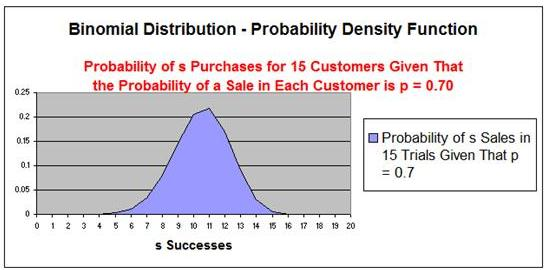Problem 6

Problem 6: Finding the probability of a stock trader being above average if the number of successful trades he has made is known and the probability of an above-average stock trader making a successful sale is known.

Problem: An above-average stock trader makes a profit in 1 out of 4 transactions. An average stock trader makes a profit on average 1 out of 7 transactions. A below-average stock trader makes a profit on 1 out of 10 transactions on average. If a stock trader conducts 15 transactions and makes a profit on 2 of them, what is the probability of the stock trader being above average?

.

Probability of Success (Profit) for Each Transaction = p = 1/4 = 0.25

Probability of No Success (No Profit) in Each Transaction = q = 1 - p = 0.75

Number of Trials (Transactions) = n = 10

Maximum Number of Successes = k = 2

This problem uses Binomial formulas (Sample Occurrence Formulas) because what is being measured is the mean number of occurrences from a large number of individual samples that each have only two possible outcomes.

Probability of making up to 2 Profitable Transactions = Pr(X=1) + Pr(X=2)

PR (X = 1) = f(k; n, p) = n! / [ k! * ( n - k )! ] * pk * q(n-k)

PR (X = 1) = f(1; 10, 0.25) = 1! / [ 10! * ( 10 - 1 )! ] * (0.25)\1 * (0.75)(10-1)

PR (X = 1) = 0.188 = 18.8%

PR (X = 2) = f(k; n, p) = n! / [ k! * ( n - k )! ] * pk * q(n-k)

PR (X = 2) = f(2; 10, 0.25) = 2! / [ 10! * ( 10 - 2 )! ] * (0.25)2 * (0.75)(10-2)

PR (X = 2) = 0.281 = 28.1%

Pr(X=1) + Pr(X=2)  = 18.8% + 28.1% = 46.9%

There is a 46.9% probability that the stock trader is above average if he completes up to 2 successful transactions in his next 10 attempts if the above average stock trader normally completes 1 out of 4 transactions profitably.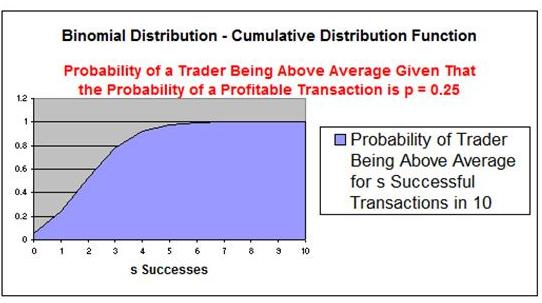Problem 7

Problem 7: Finding the probability of a certain type of package passing down a conveyor belt if the probability of that type of package passing by is known.

Problem: A conveyor belt brings packages to a truck to loaded. The packages are either black or white. The probablity that a package is black is 40%. What is the probability that out of the next 10 packages, at least 2 are black and 2 are white?

The only possibility of at least two packages being white and 2 black would occur if the number of black packages equaled 0, 1, 9, or 10. The probability of at least 2 packages being black and 2 white would therefore equal 1 minus the probability that the number of black packages equals 0, 1, 9, or 10.

Probability of Success (Black Package) = p = 0.40

Probability of No Success (White Package) = q = 1 - p = 0.60

Number of Trials (Transactions) = n = 10

Exact Number of Successes = k = 0, 1, 9, 10

This problem uses Binomial formulas (Sample Occurrence Formulas) because what is being measured is the mean number of occurrences from a large number of individual samples that each have only two possible outcomes.

Probability of at least 2 Black and 2 White Packages in 10 =

1 - [ Pr(X=0) + Pr(X=1) + Pr(9) + Pr(10) ]

PR (X = 0) = f(k; n, p) = n! / [ k! * ( n - k )! ] * pk * q(n-k)

PR (X = 0) = f(0; 10, 0.40) = 0! / [ 10! * ( 10 - 0 )! ] * (0.40)\0 * (0.60)(10-0)

PR (X = 0) = 0.0060 = 0.60%

PR (X = 1) = f(k; n, p) = n! / [ k! * ( n - k )! ] * pk * q(n-k)

PR (X = 1) = f(1; 10, 0.40) = 1! / [ 10! * ( 10 - 1 )! ] * (0.40)\1 * (0.60)(10-1)

PR (X = 1) = 0.040 = 4.0%

PR (X = 9) = f(k; n, p) = n! / [ k! * ( n - k )! ] * pk * q(n-k)

PR (X = 9) = f(9; 10, 0.40) = 9! / [ 10! * ( 10 - 9 )! ] * (0.40)\9 * (0.60)(10-9)

PR (X = 9) = 0.002 = 0.2%

PR (X = 10) = f(k; n, p) = n! / [ k! * ( n - k )! ] * pk * q(n-k)

PR (X = 10) = f(10; 10, 0.40) = 10! / [ 10! * ( 10 - 10 )! ] * (0.40)10 * (0.60)(10-10)

PR (X = 10) = 0.0 = 0.0%

1 - [ Pr(X=0) + Pr(X=1) + Pr(9) + Pr(10) ]  =  1 - [ 0.006 + 0.040 + 0.002 + 0.0 ]

= 1 -  0.048 = 0.952
= 95.2%

There is a 95.2% probability at at least 2 packages will be black and 2 packages will be white out of the next 10 packages if the probability of a package being black is 40%.

Problem 8

Problem 8: Finding the probability of getting a 4 at least twice in 5 tosses of a pair of fair dice.

Problem: Find the probability of getting a 4 at least twice in 5 tosses of a pair of fair dice. A fair die has a probability of 1/6 of landing on any of the 6 possible sides.

.A pair of dice can have 36 possible combinations (62). Four of those combinations can add up to 4 [ (1,3) (2,2) (3,1) (2,2) ]. The probability of getting a 4 in one roll of a pair of dice is 4/36 = .111 = 11.1%

Probability of Success (Getting a 4) in any Roll = p = 11.1 = .111

Probability of No Success (Not getting a 4) in any Roll = q = 1 - p = .889

Number of Trials (Rolls of a pair of dice) = n = 5

Exact Number of Successes = k = 2

This problem uses Binomial formulas (Sample Occurrence Formulas) because what is being measured is the mean number of occurrences from a large number of individual samples that each have only two possible outcomes.

Probability of getting two 4's = Pr(X=2)

PR (X = 2) = f(k; n, p) = n! / [ k! * ( n - k )! ] * pk * q(n-k)

PR (X = 2) = f(2; 5, 0.111) = 5! / [ 2! * ( 5 - 2 )! ] * (0.111)2 * (0.889)(5-2)

PR (X = 2) = 0.867 = 86.7%

There is a 86.7% probability of getting a 4 at least twice in 5 tosses of a pair of fair dice.

If You Like This, Then Share It...